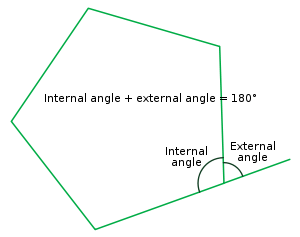# Internal and external angles

"Interior angle" redirects here. For interior angles on the same side of the transversal, see Transversal line.
 Basic Angle Types Types of Angles 2D Angles 2D Angle Pairs 3D Angles DihedralInternal and External angles

In geometry, an angle of a polygon is formed by two sides of the polygon that share an endpoint. For a simple (non-self-intersecting) polygon, regardless of whether it is convex or non-convex, this angle is called an interior angle (or internal angle) if a point within the angle is in the interior of the polygon. A polygon has exactly one internal angle per vertex.

If every internal angle of a simple polygon is less than 180°, the polygon is called convex.

In contrast, an exterior angle (or external angle) is an angle formed by one side of a simple polygon and a line extended from an adjacent side.:pp. 261-264

• The sum of the internal angle and the external angle on the same vertex is 180°.
• The sum of all the internal angles of a simple polygon is 180(n–2)° where n is the number of sides. The formula can be proved using mathematical induction and starting with a triangle for which the angle sum is 180°, then replacing one side with two sides connected at a vertex, and so on.
• The sum of the external angles of any simple convex or non-convex polygon is 360°.
• The measure of the exterior angle at a vertex is unaffected by which side is extended: the two exterior angles that can be formed at a vertex by extending alternately one side or the other are vertical angles and thus are equal.

The interior angle concept can be extended in a consistent way to crossed polygons such as star polygons by using the concept of directed angles. In general, the interior angle sum in degrees of any closed polygon, including crossed (self-intersecting) ones, is then given by 180(n–2k)° where n is the number of vertices and the non-negative number k is the number of total revolutions of 360° one undergoes walking around the perimeter of the polygon. In other words, 360k° represents the sum of all the exterior angles. For example, for ordinary convex and concave polygons k = 1, since the exterior angle sum is 360°, and one undergoes only one full revolution walking around the perimeter.

## References

1. Weisstein, Eric W. "Exterior Angle Bisector." From MathWorld--A Wolfram Web Resource. http://mathworld.wolfram.com/ExteriorAngleBisector.html
2. Posamentier, Alfred S., and Lehmann, Ingmar. The Secrets of Triangles, Prometheus Books, 2012.